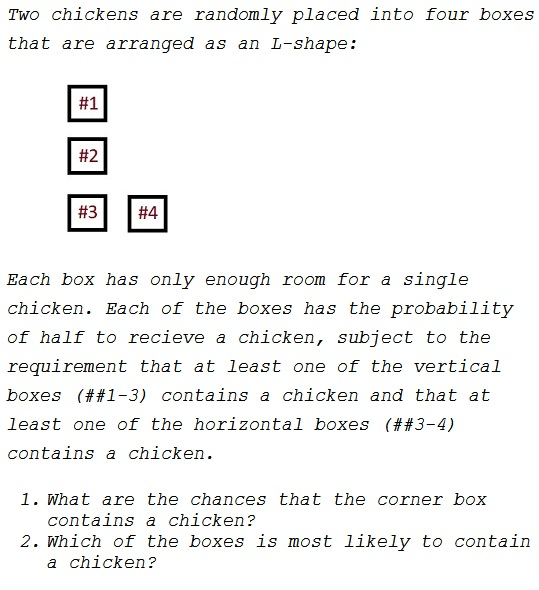# Two Chickens in Boxes

### Problem### Solution 1

As in the earlier problem, there are $16$ equiprobable chicken placements, of which five satisfy the conditions of the problem:

$\begin{array}{ccccc} \textit{Box #}&1&2&3&4\\ &\text{chicken}&&&\text{chicken}\\ &&\text{chicken}&&\text{chicken}\\ &&&\text{chicken}&\text{chicken}\\ &\text{chicken}&&\text{chicken}\\ &&\text{chicken}&\text{chicken}\\ \end{array}$

Thus boxes #1-2 contain a chicken with the probability $\displaystyle \frac{2}{5};$ for boxes #3-4, the probability is $\displaystyle \frac{3}{5}.$

### Solution 2

Chicken placements CCxx, CxCx, CxxC, xCCx, xCxC, xxCC are equally likely. It follows that prior Odds in box 3 are $1:1,$ $Pr("yes"|\text{in box 3})=1,$ $Pr("yes"|\text{not in box 3})=2/3$ and posterior odds are $3/2:1,$ or $3:2,$ i.e. $Pr(\text{in box 3})=3/5.$

### Acknowledgment

This is a modification of a problem from P. J. Nahin's Will You Be Alive 10 Years from Now? (Princeton University Press, 2014).

Solution 2 is by Joshua Miller.